## Cipher## Dec 25, 2016

### AstroNOT Piers Sellers dies at 61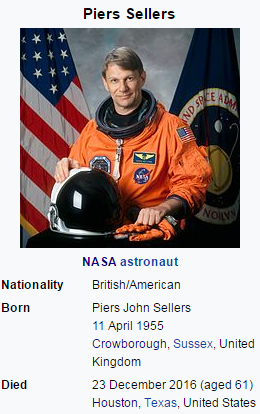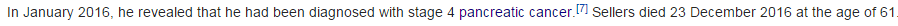Died 11 months after revealing cancer in JanuaryNinety = 5+9+5+5+2+7 = 33
27 in Decimal system is 33 in Octal numbering system

He was born on 4/11/1955 = 4+1+1+1+9+5+5 = 26 (Cancer = 26 E. Reduction), Mason = 26, Lie = 26, God = 26

Died - 12/23/2016 = 1+2+2+3+2+0+1+6 = 17 (Kill - 17, Space - 17)
12/23/2016 = 12+23+2+0+1+6 = 44 (Cancer = 44, Kill = 44, Space = 44) he was born on 4/11 (4x11 = 44)
12/23/2016 = 12+23+20+16 = 71 (Pancreatic cancer = 71)
12/23/16 = 12+23+16 = 51 (Conspiracy = 51, he was chosen for NASA Group 16 on 5/1/1996)

December = 55, he was born in 1955,  he died on 257th day after his birthday this year (55th prime number is 257), Sacrifice = 55 (S exception)On October 21st this year was released a documentary film called "Before the flood"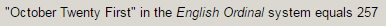October = 6+3+2+6+2+5+9 = 33
Released - 10/21/16 = 10+21+16 = 47 (his middle name "John" = 47, Foundation = 47, Authority = 47, Obey = 47, Time = 47, Agent = 47, Star of David = 47)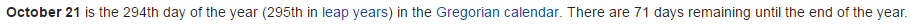October 21st leaves 71 days in the year, Pancreatic cancer = 71, and he died on a date numerology of 71Director of "Earth Science"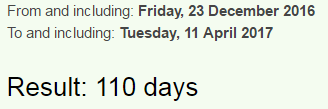He died 110 days before his next birthday